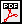Module 26 - Activities for Calculus Using the TI-89 Introduction | Assignment | Activity 1 | Activity 2 | Activity 3 | Activity 4 | Activity 5 Activity 1: Infinite Series - Fractals Overview: Fractal geometry provides a new way for mathematicians and scientists to explore nature. Euclidean geometry models many regularly shaped natural phenomena such as cubic crystals, spherical planets, and elliptic orbits. Fractal geometry models irregular objects such as coastlines, mountains, clouds, plants, and the human brain. Models in nature are only finite approximations of fractals. A fractal is a self-similar geometric figure resulting from beginning with an initial figure and iterating a process an infinite number of times. This procedure is called recursion. A fractal has irregular (rough, crinkled) edges and fractal (fractional) dimension. Materials: TI-89 Activity:Infinite Series - Fractals < Back | Next > ©Copyright 2007 All rights reserved. | Trademarks | Privacy Policy | Link Policy